21

10

2

3

# MDBM.jlMulti-Dimensional Bisection Method (MDBM) is an efficient and robust root-finding algorithm, which can be used to determine whole high-dimensional submanifolds (points, curves, surfaces…) of the roots of implicit non-linear equation systems, especially in cases, where the number of unknowns surpasses the number of equations.

This type of problems can be found in many different field of science, just to mention a few:

• differential geometry (isolines, isosurfaces in higher dimensions)
• analysis of linkages (mechanical: workspace of robots)
• stability computation, stabilyzability diagrams

This method is an alternative to the contour plot or to isosurfaces in higher dimension, however, it has as the advantage of being able to handle multiple functions at once.
In addition, it uses far less function evaluation than the brute-force approach, making it much faster and more memory efficient, especially for complex tasks.

## Introduction

The bisection method - or the so-called interval halving method - is one of the simplest root-finding algorithms which is used to find zeros of continuous non-linear functions. This method is very robust and it always tends to the solution if the signs of the function values are different at the borders of the chosen initial interval.

Geometrically, root-finding algorithms of f(x)=0 find one intersection point of the graph of the function with the axis of the independent variable. In many applications, this 1-dimensional intersection problem must be extended to higher dimensions, e.g.: intersections of surfaces in a 3D space (volume), which can be described as a system on non-linear implicit equations. In higher dimensions, the existence of multiple solutions becomes very important, since the intersection of two surfaces can create multiple intersection curves.

MDBM algorithm can handle:

• multiple solutions
• arbitrary number of variable (typically: 3-6)
• arbitrary number of implicit equations
• multiple constraints in the parameter space
• degenerated functions

while providing the gradients of the equations for the roots by means of first order interpolation (and convergence rate).

## Citing

The software in this ecosystem was developed as part of academic research. If you use the MDBM.jl package as part of your research, teaching, or other work, I would be grateful if you could cite my corresponding publication: https://pp.bme.hu/me/article/view/1236/640

## Web:

https://www.mm.bme.hu/~bachrathy/research_EN.html

# Quick start

Preparation

using MDBM

using PyPlot;
pygui(true);


## Example 1

Computation of a circle section defined by the implicit equation foo(x,y) == 0 and by the constraint c(x,y) > 0

function foo(x,y)
x^2.0+y^2.0-2.0^2.0
end
function c(x,y) #only the c>0 domain is analysed
x-y
end

ax1=Axis([-5,-2.5,0,2.5,5],"x") # initial grid in x direction
ax2=Axis(-5:2:5.0,"y") # initial grid in y direction

mymdbm=MDBM_Problem(foo,[ax1,ax2],constraint=c)
iteration=5 #number of refinements (resolution doubling)
solve!(mymdbm,iteration)

#points where the function foo was evaluated
x_eval,y_eval=getevaluatedpoints(mymdbm)

#interpolated points of the solution (approximately where foo(x,y) == 0 and c(x,y)>0)
x_sol,y_sol=getinterpolatedsolution(mymdbm)

fig = figure(1);clf()
scatter(x_eval,y_eval,s=5)
scatter(x_sol,y_sol,s=5)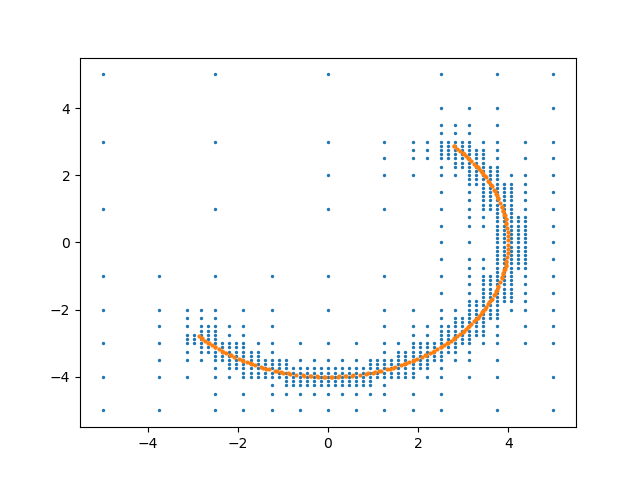Perform the line connection

myDT1=connect(mymdbm);
for i in 1:length(myDT1)
dt=myDT1[i]
P1=getinterpolatedsolution(mymdbm.ncubes[dt],mymdbm)
P2=getinterpolatedsolution(mymdbm.ncubes[dt],mymdbm)
plot([P1,P2],[P1,P2], color="k")
end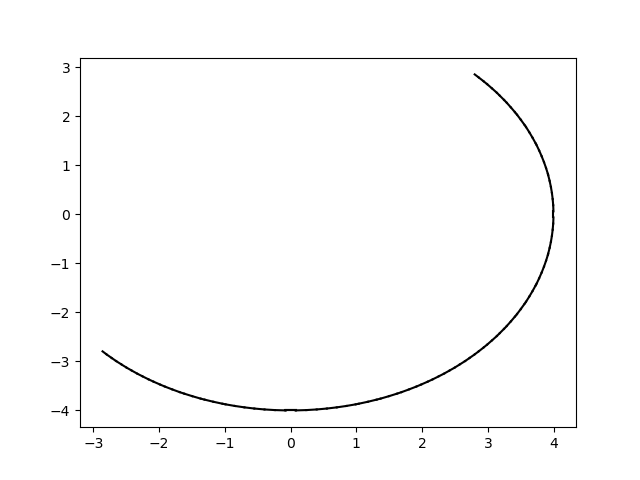## Example 2

Intersection of two sphere in 3D

using LinearAlgebra

axes=[-2:2,-2:2,-2:2]

fig = figure(3);clf()

#Sphere1
fS1(x...) = norm(x,2.0)-1

Sphere1mdbm=MDBM_Problem(fS1,axes)
solve!(Sphere1mdbm,4)
a_sol,b_sol,c_sol=getinterpolatedsolution(Sphere1mdbm)
plot3D(a_sol,b_sol,c_sol,linestyle="", marker=".", markersize=1);

#Sphere2
fS2(x...) = norm(x .- 0.5, 2.0) -1.0

Sphere2mdbm=MDBM_Problem(fS2,axes)
solve!(Sphere2mdbm,4)
a_sol,b_sol,c_sol=getinterpolatedsolution(Sphere2mdbm)
plot3D(a_sol,b_sol,c_sol,linestyle="", marker=".", markersize=1);

#Intersection
fS12(x...) = (fS1(x...), fS2(x...))

Intersectmdbm=MDBM_Problem(fS12,axes)
solve!(Intersectmdbm,6)
a_sol,b_sol,c_sol=getinterpolatedsolution(Intersectmdbm)
plot3D(a_sol,b_sol,c_sol,color="k",linestyle="", marker=".", markersize=2);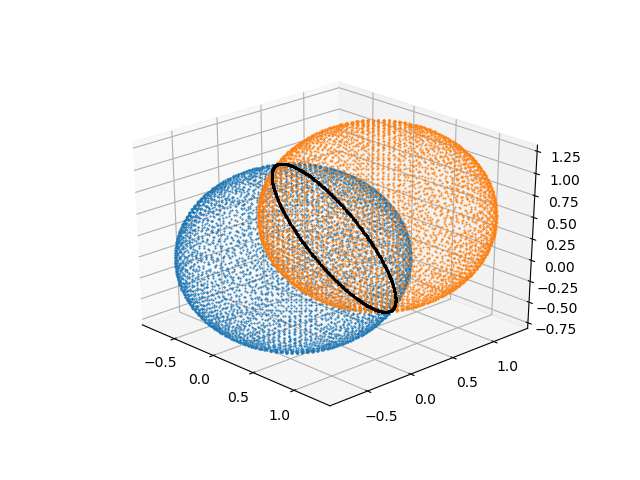## Example 3

Mandelbrot set (example for a non-smooth problem)

function mandelbrot(x,y)
c=x+y*im
z=Complex(zero(c))
k=0
maxiteration=1000
while (k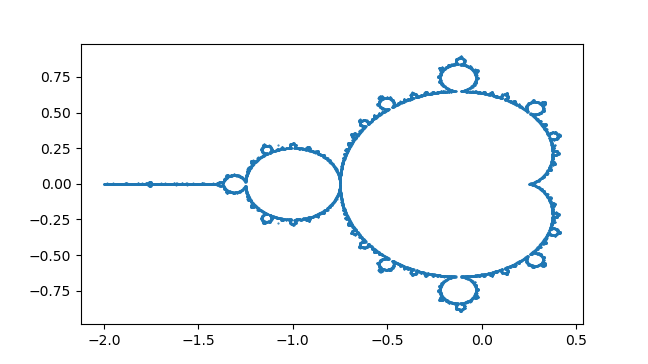History I am an assistant professor at the Budapest University of Technology and Economics, at the Faculty of Mechanical Engineering, the Department of Applied Mechanics. During my studies and research, I have to determine stability charts of models described by delayed differential equations, which are typically formed as a "3 parameter / 2 implicit equation" problem. I have faced the difficulty that there is no applicable solution in any available software (e.g.: Mathematica, Matlab,...) which could easily be used in engineering problems. Due to this reason, I have started to develop the Multi-Dimensional Bisection Method since 2006 in Matlab, and I have been improving it since, by adding new features from time to time. I hope that others also find this package a useful tool in their work. Best regards, Dr. Daniel Bachrathy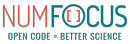MDBM is a fiscally sponsored project of NumFOCUS, a nonprofit dedicated to supporting the open source scientific computing community. 
 
 First Commit 08/09/2018 Last Touched 6 days ago Commits 46 commits Requires: Used By: $(document).ready(function() {$(".cs-secondary-checkbox[data-toggle='tooltip']").tooltip(); }); $(".cs-require-panels .checkbox:not(.disabled)").click(function() { var includeIndirectDependencies = this.getElementsByTagName("input").checked; if ( includeIndirectDependencies == true ) { this.parentElement.parentElement.classList.remove("cs-hide-indirect-dependencies"); } else { this.parentElement.parentElement.classList.add("cs-hide-indirect-dependencies"); } });$(".cs-require-panels .list-group").on('mousewheel DOMMouseScroll', function (e) { var e0 = e.originalEvent; var delta = e0.wheelDelta || -e0.detail; if ( delta < 0 ) { return; } this.scrollTop -= 30; e.preventDefault(); }); Julia Observer 
 Pkg.add("MDBM") Activity Loading... new Chartkick["AreaChart"]("chart-1", [[1,0],[2,0],[3,0],[4,0],[5,0],[6,0],[7,0],[8,0],[9,0],[10,0],[11,0],[12,0],[13,0],[14,0],[15,0],[16,0],[17,0],[18,0],[19,0],[20,0],[21,0],[22,0],[23,0],[24,0],[25,0],[26,0],[27,0],[28,0],[29,0],[30,0],[31,0],[32,0],[33,0],[34,0],[35,0],[36,0],[37,0],[38,0],[39,0],[40,0],[41,0],[42,0],[43,0],[44,0],[45,0],[46,0],[47,0],[48,0],[49,0],[50,0],[51,0],[52,0]], {"xtitle":"Week","ytitle":"Commits"}); Contributors Versions Julia Observer $(document).ready(function(){ var clipboard = new Clipboard('.js-copy-button'); var curRipple =$(".js-ripple"), newHeight = curRipple.parent().outerHeight(); curRipple.height(newHeight); // copy pasta [beg] (function (window, $) {$(function() { $('.js-ripple').on('click', function (event) { event.preventDefault(); var curDiv =$('<div/>'), btnOffset = $(this).offset(), xPos = event.pageX - btnOffset.left, yPos = event.pageY - btnOffset.top; curDiv.addClass('ripple-effect'); var ripple =$(".ripple-effect"); ripple.css("height", $(this).height()); ripple.css("width",$(this).height()); curDiv .css({ top: yPos - (ripple.height()/2), left: xPos - (ripple.width()/2), background: $(this).data("ripple-color") }) .appendTo($(this)); window.setTimeout(function(){ curDiv.remove(); }, 2000); }); }); })(window, jQuery); // copy pasta [end] });